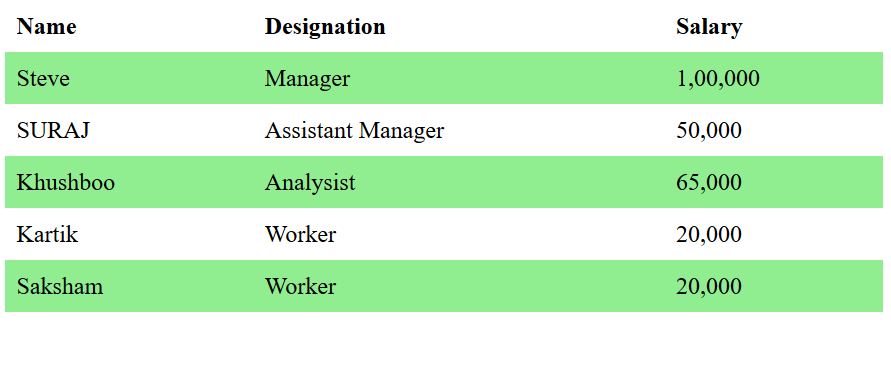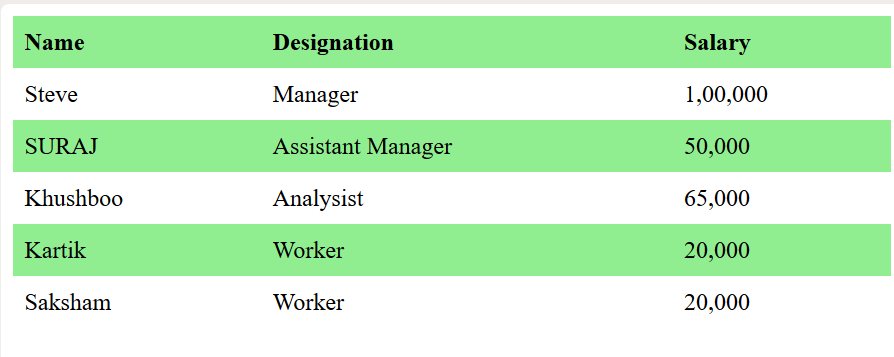# How to set alternate table row color using CSS?

The :nth-child() selector in CSS is used to match the elements based on their position in a group of siblings. It matches every element that is the nth child.

Syntax:

```:nth-child(number) {
// CSS Property
}```

Where number is the argument that represents the pattern for matching elements. It can be odd, even or in a functional notation.

• odd: It represents the elements whose position is odd in a series: 1, 3, 5, etc.

Syntax:

`element:nth-child(even)`
• even: It represents the elements whose position is even in a series: 2, 4, 6, etc.

Syntax:

`element:nth-child(odd)`

Example 1: It sets the color to alternate even rows in a table.

 ` ` `<``html``> ` `     `  `<``head``> ` `     `  `    ``` `    ``<``style``> ` `        ``table { ` `            ``border-collapse: collapse; ` `            ``width: 100%; ` `        ``} ` `         `  `        ``th, td { ` `            ``text-align: left; ` `            ``padding: 8px; ` `        ``} ` `         `  `        ``tr:nth-child(even) { ` `            ``background-color: Lightgreen; ` `        ``} ` `    `` ` ` ` ` `  `<``body``> ` `    ``<``table``> ` `        ``<``tr``> ` `            ``<``th``>Name ` `            ``<``th``>Designation ` `            ``<``th``>Salary ` `        `` ` `         `  `        ``<``tr``> ` `            ``<``td``>Steve ` `            ``<``td``>Manager ` `            ``<``td``>1,00,000 ` `        `` ` `         `  `        ``<``tr``> ` `            ``<``td``>SURAJ ` `            ``<``td``>Assistant Manager ` `            ``<``td``>50,000 ` `        `` ` `         `  `        ``<``tr``> ` `            ``<``td``>Khushboo ` `            ``<``td``>Analysist ` `            ``<``td``>65,000 ` `        `` ` `         `  `        ``<``tr``> ` `            ``<``td``>Kartik ` `            ``<``td``>Worker ` `            ``<``td``>20,000 ` `        `` ` `         `  `        ``<``tr``> ` `            ``<``td``>Saksham ` `            ``<``td``>Worker ` `            ``<``td``>20,000 ` `        `` ` `    `` ` ` ` ` `  ` `

Output:Example 2: It sets the color to alternate odd rows in a table.

 ` ` `<``html``> ` `     `  `<``head``> ` `    ``<``title``> ` `        ``Set alternate row in table ` `    `` ` `     `  `    ``<``style``> ` `        ``table { ` `            ``border-collapse: collapse; ` `            ``width: 100%; ` `        ``} ` `         `  `        ``th, td { ` `            ``text-align: left; ` `            ``padding: 8px; ` `        ``} ` `         `  `        ``tr:nth-child(odd) { ` `            ``background-color: Lightgreen; ` `        ``} ` `    `` ` ` ` ` `  `<``body``> ` `    ``<``table``> ` `        ``<``tr``> ` `            ``<``th``>Name ` `            ``<``th``>Designation ` `            ``<``th``>Salary ` `        `` ` `         `  `        ``<``tr``> ` `            ``<``td``>Steve ` `            ``<``td``>Manager ` `            ``<``td``>1, 00, 000 ` `        `` ` `         `  `        ``<``tr``> ` `            ``<``td``>SURAJ ` `            ``<``td``>Assistant Manager ` `            ``<``td``>50, 000 ` `        `` ` `         `  `        ``<``tr``> ` `            ``<``td``>Khushboo ` `            ``<``td``>Analysist ` `            ``<``td``>65, 000 ` `        `` ` `         `  `        ``<``tr``> ` `            ``<``td``>Kartik ` `            ``<``td``>Worker ` `            ``<``td``>20, 000 ` `        `` ` `         `  `        ``<``tr``> ` `            ``<``td``>Saksham ` `            ``<``td``>Worker ` `            ``<``td``>20, 000 ` `        `` ` `    `` ` `     ` ` `  `<``html``> `

Output:My Personal Notes arrow_drop_upCheck out this Author's contributed articles.

If you like GeeksforGeeks and would like to contribute, you can also write an article using contribute.geeksforgeeks.org or mail your article to contribute@geeksforgeeks.org. See your article appearing on the GeeksforGeeks main page and help other Geeks.

Please Improve this article if you find anything incorrect by clicking on the "Improve Article" button below.

Article Tags :

Be the First to upvote.

Please write to us at contribute@geeksforgeeks.org to report any issue with the above content.